## Array

An array is a list of lists'' with the length of each level of list the same. The size (sometimes called the shape'') of a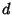-dimensional array is then indicated as. The most common type of array encountered is the 2-D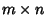rectangular array havingcolumns androws. If, a square array results. Sometimes, the order of the elements in an array is significant (as in a Matrix), whereas at other times, arrays which are equivalent modulo reflections (and rotations, in the case of a square array) are considered identical (as in a Magic Square or Prime Array).

In order to exhaustively list the number of distinct arrays of a given shape with each element being one ofpossible choices, the naive algorithm of running through each case and checking to see whether it's equivalent to an earlier one is already just about as efficient as can be. The running time must be at least the number of answers, and this is so close tothat the difference isn't significant.

However, finding the number of possible arrays of a given shape is much easier, and an exact formula can be obtained using the Polya Enumeration Theorem. For the simple case of anarray, even this proves unnecessary since there are only a few possible symmetry types, allowing the possibilities to be counted explicitly. For example, consider the case ofandEven and distinct, so only reflections need be included. To take a specific case, let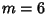andso the array looks like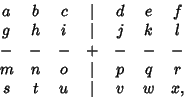where each,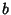, ...,can take a value from 1 to. The total number of possible arrangements is(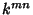in general). The number of arrangements which are equivalent to their left-right mirror images is(in general,), as is the number equal to their up-down mirror images, or their rotations through 180°. There are also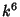arrangements (in general,) with full symmetry.

In general, it is therefore true thatso there arearrangements with no symmetry. Now dividing by the number of images of each type, the result, forwithEven, is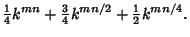The number is therefore of order, with correction'' terms of much smaller order.

See also Antimagic Square, Euler Square, Kirkman's Schoolgirl Problem, Latin Rectangle, Latin Square, Magic Square, Matrix, Mrs. Perkins' Quilt, Multiplication Table, Orthogonal Array, Perfect Square, Prime Array, Quotient-Difference Table, Room Square, Stolarsky Array, Truth Table, Wythoff Array